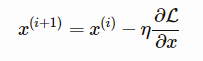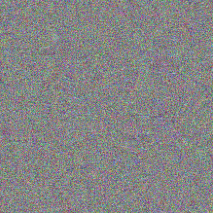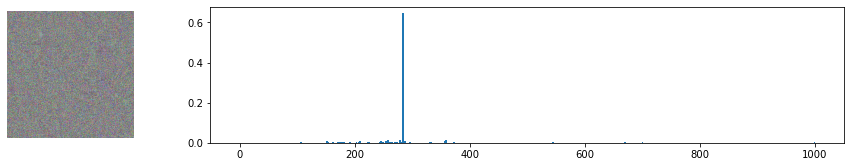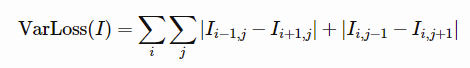# How a Neural Network Sees a Cat

Deep LearningModelingMicrosoftposted by ODSC Community July 12, 2022

Article by Carlotta Castelluccio and Dmitry Soshnikov of Microsoft. As human beings, we know what a cat looks like. But what...Article by Carlotta Castelluccio and Dmitry Soshnikov of Microsoft.

As human beings, we know what a cat looks like. But what about neural networks? In this post, we reveal what a cat looks like inside a neural network brain, and also talk about adversarial attacks.

Real and Ideal cat. Image on the left is from Unsplash

Convolutional Neural Networks work great for image classification. There are many pre-trained networks, such as VGG-16 and ResNet, trained on the ImageNet dataset, that can classify an image into one of 1000 classes. The way they work is to learn patterns that are typical for different object classes, and then look through the image to recognize those classes and take the decision. It is similar to a human being, who is scanning a picture with his/her eyes, looking for familiar objects.

## AI for Beginners Curriculum

If you want to learn more about convolutional neural networks, and about neural networks in general — we recommend you to visit AI for Beginners Curriculum, available on Microsoft’s GitHub. It is a collection of learning materials organized into 24 lessons, which can be used by students/developers to learn about AI, and by teachers, who might find useful materials to include in their classes. This blog post is based on materials from the curriculum.So, once trained, a neural network contains different patterns inside its brain, including notions of the ideal cat (as well as an ideal dog, ideal zebra, etc.). However, it is not very easy to visualize those images, because patterns are spread all over the network weights, and also organized in a hierarchical structure. Our goal would be to visualize the image of an ideal cat that a neural network has inside its brain.

## Classifying Images

Classifying images using a pre-trained neural network is simple. Using Keras, we can load a pre-trained model with one line of code:

```model =
keras.applications.VGG16(weights='imagenet',include_top=True
)```

For each input image of size 224x224x3, the network will give us a 1000-dimensional vector of probabilities, each coordinate of this vector corresponding to a different ImageNet class. If we run the network on the noise image, we will get the following result:

```x = tf.Variable(tf.random.normal((1,224,224,3)))
plot_result(x)```

You can see that there is one class with a higher probability than the others. In fact, this class is mosquito net, with a probability of around 0.06. Indeed, random noise looks similar to a mosquito net, but the network is still very unsure, giving many other options!

# Optimizing for a Cat

Our main idea to obtain the image of an ideal cat is to use gradient descent optimization technique to adjust our original noisy image in such a way that the network starts thinking it’s a cat.

Optimization loop to obtain images that are classified as a cat

Suppose that we start with the original noise image x. VGG network gives us some probability distribution V(x). To compare it to the desired distribution of a cat, we can use cross-entropy loss function, and calculate the loss L = cross_entropy_loss(c,V(x)).

To minimize the loss, we need to adjust our input image. We can use the same idea of gradient descent that is used for the optimization of neural networks. Namely, at each iteration, we need to adjust the input image x according to the formula:Here η is the learning rate, which defines how radical our changes to the image will be. The following function will do the trick:

```target =  # Siamese cat

defcross_entropy_loss(target,res):
return tf.reduce_mean(
keras.metrics.sparse_categorical_crossentropy(target,res))

defoptimize(x,target,loss_fn, epochs=1000, eta=1.0):
for i in range(epochs):
res = model(x)
loss = loss_fn(target,res)

optimize(x,target,cross_entropy_loss)```As you can see, we got something very similar to random noise. This is because there are many ways to make the network think the input image is a cat, including some that do not make sense visually. While those images contain a lot of patterns typical for a cat, there is nothing to constrain them to be visually distinctive. However, if we try to pass this ideal noisy cat to the VGG network, it will tell us that the noise is actually a cat with quite a high probability (above 0.6):This approach can be used to perform so-called adversarial attacks on a neural network. In an adversarial attack, our goal is to modify an image a little bit to fool a neural network, for example, make a dog look like a cat. A classical adversarial example from this paper by Ian Goodfellow looks like this:

Image from the paper Goodfellow, I.J.; Shlens, J.; Szegedy, C. Explaining and harnessing adversarial examples. arXiv 2014, arXiv:1412.6572

In our example, we will take a slightly different route, and instead of adding some noise, we will start with an image of a dog (which is indeed recognized by a network as a dog), and then tweak it a little but using the same optimization procedure as above, until the network starts classifying it as a cat:

```img = Image.open('images/dog.jpg').resize((224,224))
x = tf.Variable(np.array(img))
optimize(x,target,cross_entropy_loss)```

Below you can see the original picture (classified as Italian Greyhound with a probability of 0.93), and the same picture after optimization (classified as Siamese cat with a probability of 0.87).Original picture of a dog (from Unsplash, on the left) and a picture of a dog classified as a cat (on the right)

## Making Sense of Noise

While adversarial attacks are interesting in their own right, we have not yet been able to visualize the notion of the ideal cat that a neural network has. The reason the ideal cat we have obtained looks like noise is that we have not put any constraints on our image x that is being optimized. For example, we may want to constrain the optimization process so that the image x is less noisy, which will make some visible patterns more noticeable.

In order to do this, we can add another term to the loss function. A good idea would be to use so-called variation loss, a function that shows how similar neighboring pixels of the image are. For an image I, it is defined asTensorFlow has a built-in function `tf.image.total_variation` that computes the total variation of a given tensor. Using it, we can define our total loss function in the following way:

```deftotal_loss(target,res):
return 0.005*tf.image.total_variation(x,res) +\
10*tf.reduce_mean(sparse_categorical_crossentropy(target,res))```

Note the coefficients `0.005` and `10` – those are determined by trial-and-error to find a good balance between smoothness and detail in the image. You may want to play a bit with those to find a better combination.

Minimizing variation loss makes the image smoother, and gets rid of noise — thus revealing more visually appealing patterns. Here is an example of such “ideal” images, that are classified as cat and as zebra with high probability:

```optimize(x,,loss_fn=total_loss) # cat
optimize(x,,loss_fn=total_loss) # zebra```Ideal Cat, probability=0.92 (on the left) and Ideal Zebra, probability=0.89 (on the right)

## Takeaway

Those images give us some insights into how neural networks make sense of images. In the “cat” image, you can see some of the elements that resemble a cat’s eyes, and some of them resemble ears. However, there are many of them, and they are spread all over the image. Recall that a neural network in its essence calculates a weighted sum of its inputs, and when it sees a lot of elements that are typical for a cat — it becomes more convinced that it is in fact a cat. That’s why many eyes on one picture give us a higher probability than just one, even though it looks less “cat-like” to a human being.

## Play with the Code

Adversarial attacks and visualization of the “ideal cat” are described in the transfer learning section of the AI for Beginners Curriculum. The actual code I have covered in this blog post is available here.

Originally published at https://soshnikov.com on June 7, 2022.

## Co-authors:

Carlotta Castelluccio is a Cloud Advocate at Microsoft, focused on AI and ML technologies and passionate about their use in education. As a member of the Developer Relationships Academic team, she works on skilling and engaging educational communities to create and grow with Azure Cloud, by contributing to technical learning content and supporting students and educators in their learning journey with Microsoft technologies. Before joining the Cloud Advocacy team, she had been working as an Azure and AI consultant in the Microsoft Industry Solutions team, mainly involved in customer-face engagements focused on Conversational AI solutions.

Dmitry Soshnikov is a Microsoft veteran, who was with Microsoft for more than 16 years. He started as a Technical Evangelist, and in this role presented at numerous conferences, including twice being on stage with Steve Ballmer. He then worked for 2 years as a Senior Software Engineer, helping big European companies to start pilot digital transformation projects based on AI and ML. As Cloud Developer Advocate, Dmitry focuses on creating educational content and working with academic and research institutions. He is the primary author of AI for Beginners Curriculum.## ODSC Community

The Open Data Science community is passionate and diverse, and we always welcome contributions from data science professionals! All of the articles under this profile are from our community, with individual authors mentioned in the text itself.

1